# YEARFRAC Function

Return the number of days between two dates as a year fraction

## What is the YEARFRAC Function?

The YEARFRAC Function is categorized under Excel DATE/TIME functions. YEARFRAC will return the number of days between two dates as a year fraction, in decimal form, in Excel.

In financial analysis, one can use the function to, for example, calculate a portion of annual revenues.

### Formula

=YEARFRAC(start_date, end_date, [basis])

The YEARFRAC function uses the following arguments:

1. Start_date (required argument) – This is the start of the period. The function includes the start date in calculations.
2. End_date (required argument) – This is the end of the period. The function also includes the end date in calculations.
3. [basis] (optional argument) – Specifies the type of day count basis to be used.

Various possible values of [basis] and their meanings are: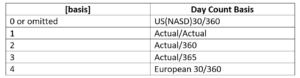### How to use the YEARFRAC Function in Excel?

As a worksheet function, YEARFRAC can be entered as part of a formula in a cell of a worksheet. To understand the uses of the function, let’s consider a few examples:

#### Example – A few simple date differences

Let’s see a few dates and how the function provides results: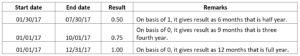The formula used was: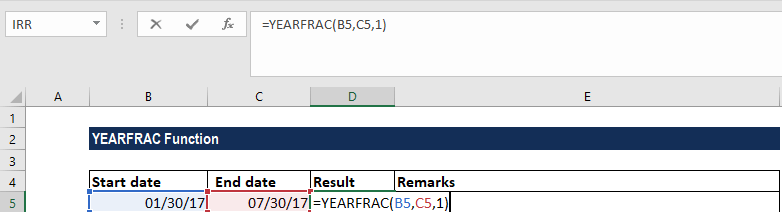The results in Excel are shown in the screenshot below: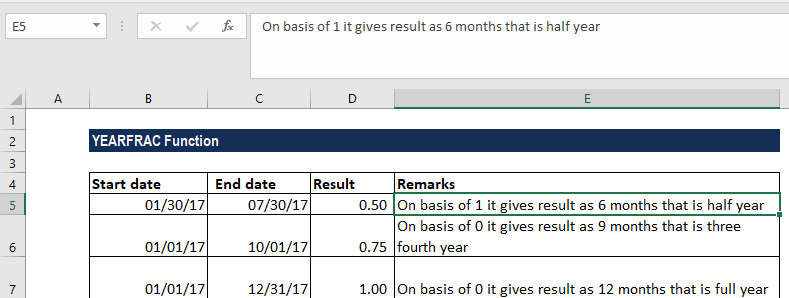#### Applied Example on how to use the YEARFRAC Function

Let’s assume we are handling the HR department and are processing data from our employee database, shown below. We realize that we have all of our employees’ dates of birth but we want to find out their age ‘value’ today. We can use the YEARFRAC function along with the INT and TODAY functions to do this. We insert a column next to the Date of Birth, label it “age”, and insert the following formula:

=INT(YEARFRAC(C5,TODAY()))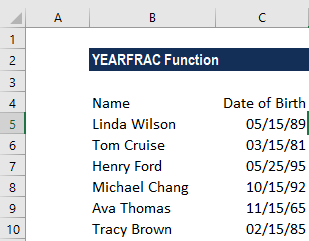The formula used looks like this: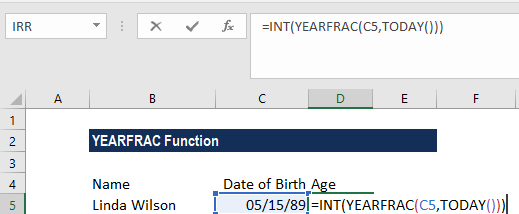The INT function will output the age value as an integer, which is the day-to-day convention. Now if you want to have a more precise value for age, such as a decimal, just remove the INT() part of the function. Excel will use whole days between two dates. As all dates are simply serial numbers, the process is straightforward in Excel.

We get the results below: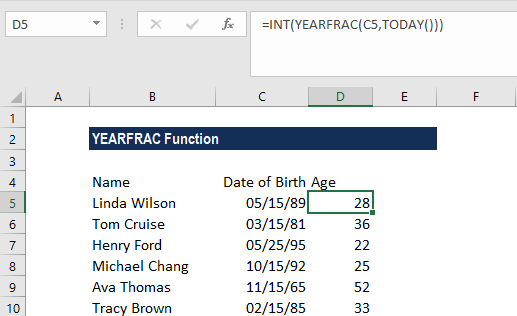If you replace the TODAY function with another date you get the age for that person on a specific date.

For example, if we are holding a competition for people aged below 35 years old, we can use the following formula:

=IF(INT(YEARFRAC(A1,TODAY()))<35,”Eligible”,”Not Eligible”)

### Things to remember about the YEARFRAC Function

1. If the dates you are using are not working, input them with the DATE() function.
2. #NUM! error – Occurs when the given basis argument is less than 0 or greater than 4.
3. #VALUE! error – Occurs when:
1. The start_date or end_date arguments are not valid dates.
2. The given [basis] argument is non-numeric.

Thanks for reading CFI’s guide to important Excel functions! By taking the time to learn and master these functions, you’ll significantly speed up your financial analysis. To learn more, check out these additional CFI resources:

• Excel Functions for Finance